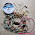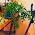MATH 1280 Introduction to Statistics – Discussion 2

Factors are qualitative data that are associated with categorization or the description of an attribute. Factors are often (but not always) represented with words, like colors or names of things.”
On the other hand, numeric data are generated by numeric measurements. R can store values as factors or numbers, but sometimes you have a choice of how to represent values.”

1) I have two apples, one banana, one cherry. Does it make sense to calculate the "average" of these things? Would you code that as a factor or a numeric value in R?
No, we cannot make average on two apples, one banana, one cherry, because they are different kind of fruits, not number.
Then, I would code Fruit as factors, since fruit is qualitative not quantitative.

2) I have four quiz scores: 94, 93, 85, and 0. What is the mean (average) of my quiz scores? Would you code this as a factor or a numeric value in R?
I use R to find out the mean (average), the result is following:
> x<-c (94, 93, 85, 0)
> mean(x)
 68
So, mean (average) = 68
Then, I have coded the four quiz scores (94, 93, 85, and 0) as numeric value in R

A mural painting
3) In another class, I received these grades on my quizzes: two As, one B, and one F. What is the mean (average) of my grades? Would you code that as a factor or a numeric value in R?
No, average is not possible here, because the letter grades are not numbers.
I would code the grades (letters) as factors in R

A colorful mural painting

4) How would you explain the difference in mean values obtained in #2 and #3 above?
We cannot make different between #2 and #3 above, since #2 is numeric whereas #3 is factor. We should convert factor to numeric first, before doing subtraction operation.

1.As usual, you really put a lot of thought and work into this post, it’s amazingly informative. Thank you for sharing this and for keeping us in the know about things some of us never consider.

Now I need to digest all that you have presented here, it’s just what many of us need. I always learn so much from your articles.

Wishing you a great weekend!

1.It is just lecture material to share to students.....

Thank you to visit my article:
MATH 1280 Introduction to Statistics – Discussion 2
@
Qualitative Vs Quantitative, credit to SlidePlayer

2.MATH 1280 Introduction to Statistics – Discussion 2
@
3) In another class, I received these grades on my quizzes: two As, one B, and one F. What is the mean (average) of my grades? Would you code that as a factor or a numeric value in R?
No, average is not possible here, because the letter grades are not numbers.
I would code the grades (letters) as factors in R

3.MATH 1280 Introduction to Statistics – Discussion 2
-
2) I have four quiz scores: 94, 93, 85, and 0. What is the mean (average) of my quiz scores? Would you code this as a factor or a numeric value in R?
I use R to find out the mean (average), the result is following:
> x<-c (94, 93, 85, 0)
> mean(x)
 68
So, mean (average) = 68
Then, I have coded the four quiz scores (94, 93, 85, and 0) as numeric value in R

2.So interesting :)

1.Thank you to visit my article:
MATH, 1280 Introduction to Statistics – Discussion 2
@
“Factors are qualitative data that are associated with categorization or the description of an attribute. Factors are often (but not always) represented with words, like colors or names of things.”

2.MATH 1280 Introduction to Statistics – Discussion 2
-
4) How would you explain the difference in mean values obtained in #2 and #3 above?
We cannot make different between #2 and #3 above, since #2 is numeric whereas #3 is factor. We should convert factor to numeric first, before doing subtraction operation.

3.I remember learning this when I was in school. So familiar!

1.Thank you to visit my article:
MATH, 1280, Introduction to Statistics – Discussion 2
@
“On the other hand, numeric data are generated by numeric measurements. R can store values as factors or numbers, but sometimes you have a choice of how to represent values.”

2.MATH 1280 Introduction to Statistics – Discussion 2
-
Illustration of Qualitative Vs Quantitative

4.This brings back memories of my grad school days taking qualitative and quantitative analysis courses.

1.I see....

Thank you to visit my article:
MATH, 1280, Introduction, to Statistics – Discussion 2
@
1) I have two apples, one banana, one cherry. Does it make sense to calculate the "average" of these things? Would you code that as a factor or a numeric value in R?
No, we cannot make average on two apples, one banana, one cherry, because they are different kind of fruits, not number.
Then, I would code Fruit as factors, since fruit is qualitative not quantitative.

2.MATH 1280 Introduction to Statistics – Discussion 2
-
“Factors are qualitative data that are associated with categorization or the description of an attribute. Factors are often (but not always) represented with words, like colors or names of things.”

5.Interesting, I remember learning the difference at one time.

1.Thank you to visit my article:
MATH, 1280, Introduction, to, Statistics – Discussion 2
@
2) I have four quiz scores: 94, 93, 85, and 0. What is the mean (average) of my quiz scores? Would you code this as a factor or a numeric value in R?
I use R to find out the mean (average), the result is following:
> x<-c (94, 93, 85, 0)
> mean(x)
 68
So, mean (average) = 68
Then, I have coded the four quiz scores (94, 93, 85, and 0) as numeric value in R

2.MATH 1280 Introduction to Statistics – Discussion 2
-
1) I have two apples, one banana, one cherry. Does it make sense to calculate the "average" of these things? Would you code that as a factor or a numeric value in R?